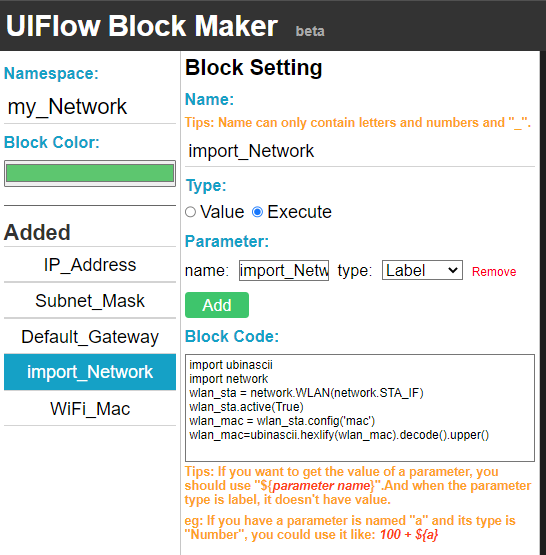• Hi,
Is there anyway to get the WiFi Mac Address for an M5stack Tough via the M5BlockMaker. I need a unique ID for the device to write in a Database.
I tried some code but always gets the devices hanging and not responding, the latest was:

import ubinascii
import network
ubinascii.hexlify(network.WLAN(network.STA_IF)).decode()

The above was obtained from: link text

• Hello @RASBR

try something like below. Works for me.

``````import network
import binascii

label0 = M5Label('label0', x=13, y=16, color=0x000, font=FONT_MONT_22, parent=None)
wlan = network.WLAN(network.STA_IF)
mac_bytes = wlan.config('mac')
mac_str = binascii.hexlify(mac_bytes).decode()
label0.set_text(mac_str)
``````

Thanks
Felix

• Hi @felmue .
Thanks for the reply. I think I got the solution the same time you sent me yours. I did use the code in here as below:

I did an 'Execute' Block:

`````` import ubinascii
import network
wlan_sta = network.WLAN(network.STA_IF)
wlan_sta.active(True)
wlan_mac = wlan_sta.config('mac')
wlan_mac=ubinascii.hexlify(wlan_mac).decode().upper()
``````then a Label Block:

``````wlan_mac
``````Anyway, thanks for taking the time and effort to reply.
Rami